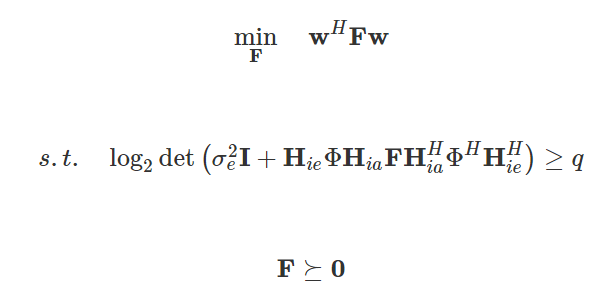# How can i use cvx to solve this SDP?I would pre-form the H_{ie} \Phi H_{ia} term, then enter the problem almost as written, except changing `log2det` to `log_det/log(2)`. You will need to declare `w` as complex, and then ^H will just be `'` , if that is indeed your intention. Of course, this presupposes you have read the CVX Users’ Guide to learn the basics of CVX.

thank, you
there exist “log_det” function in CVX users’ guide, whether you means using log_det function rather log2(det) function?

Sorry. That was a typo, which I just corrected. Use `log_det(...)/log(2)`

ok, thank you very much